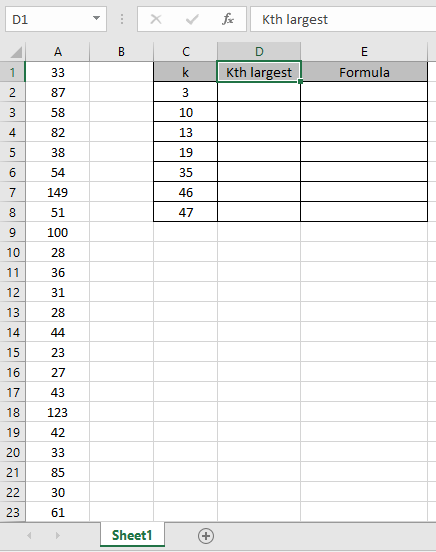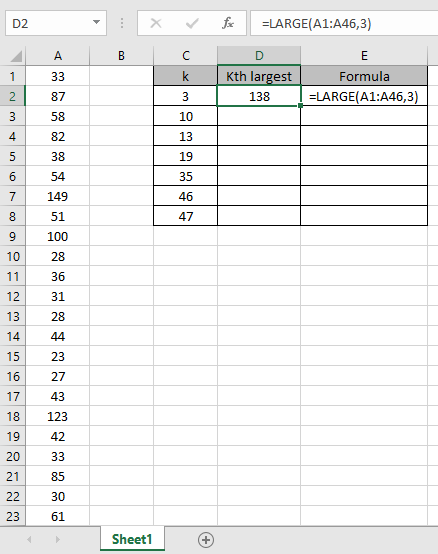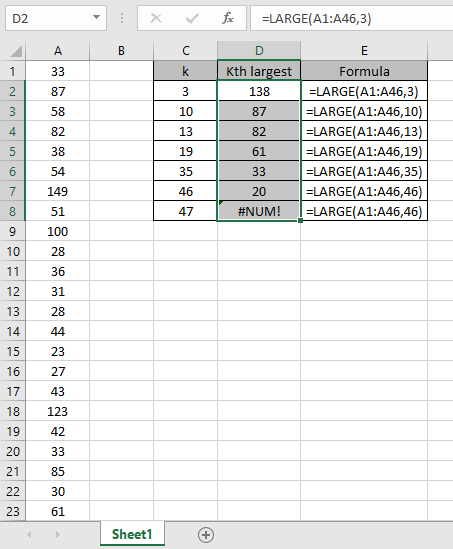# How to use LARGE function in Microsoft Excel

The LARGE function returns the kth largest value starting from the largest in a range of values.
Syntax:

=LARGE (array, k)

Note: It will return #NUM! Error if k is greater than the number of values in the range.
Let’s understand this function using it in an example.

Here we have a range of values from A1:A46 and we will apply the formula to the values to get the results for different k values.Use the formula

=LARGE (A1:A46, 3)

This function will return the 3rd largest value from the array.Now we extracted different values for different k as shown in the snapshot below.As you can see it returns #NUM! Error if k is greater than the number of values in the range.

Use the value of K = 1; for getting the MAX value and K = 46 for the MIN value. But for the MAX & MIN value use the MAX function & MIN function in excel

Hope you understood how to use LARGE function and referring cell in Excel. Explore more articles on Excel mathematical functions here. Please feel free to state your query or feedback for the above article.

Related Articles:

How to use the SMALL Function in Excel

How to Use RANK in Excel

Sort numbers using Excel SMALL function

Popular Articles:

50 Excel Shortcuts to Increase Your Productivity

How to use the VLOOKUP Function in Excel

How to use the COUNTIF in Excel 2016

How to use the SUMIF Function in Excel

Terms and Conditions of use

The applications/code on this site are distributed as is and without warranties or liability. In no event shall the owner of the copyrights, or the authors of the applications/code be liable for any loss of profit, any problems or any damage resulting from the use or evaluation of the applications/code.